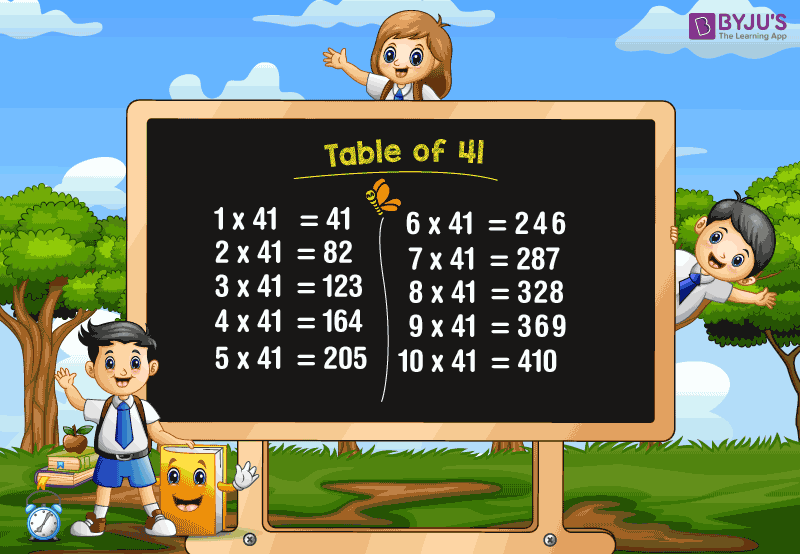# Table of 41

Table of 41 is a Maths multiplication table for the number forty-one – 41. A multiplication table is a mathematical table used to define a multiplication operation for an algebraic system in mathematics. Students can use these Tables from 1 to 100 while solving multiplication and division problems.

### Download Table of 41 HereWhat is the table of 41?

Forty-one or 41 times a number represents that 41 is repeatedly added as many times as required. For example, 41 times 5 means 41 has been added to itself five times.

i.e. 41 × 5 = 41 + 41 +41 + 41 + 41 = 205

Here, we can observe the repeated addition of 41 for 5 times.

Thus, 41 × 5 = 205

Similarly, we can find the other multiples of 41. The below table shows the multiplication of forty-one with different numbers, i.e. from 1 to 20.

## Multiplication Table of 41

 41 × 1 = 41 41 × 2 = 82 41 × 3 = 123 41 × 4 = 164 41 × 5 = 205 41 × 6 = 246 41 × 7 = 287 41 × 8 = 328 41 × 9 = 369 41 × 10 = 410 41 × 11 = 451 41 × 12 = 492 41 × 13 = 533 41 × 14 = 574 41 × 15 = 615 41 × 16 = 656 41 × 17 = 697 41 × 18 = 738 41 × 19 = 779 41 × 20 = 820

## 41 Times Table

Read the forty-one times table from 1 to 10 here.

• One time forty-one is forty one
• Two times forty-one is eighty two
• Three times forty-one is one hundred and twenty three
• Four times forty-one is one hundred and sixty four
• Five times forty-one is two hundred and five
• Six times forty-one is two hundred and forty six
• Seven times forty-one is two hundred and eighty seven
• Eight times forty-one is three hundred and twenty eight
• Nine times forty-one is three hundred and sixty nine
• Ten times forty-one is four hundred and ten

### Check More Tables

Visit www.byjus.com for more math tables and learn several other important math topics more engagingly and efficiently at BYJU’S – The Learning App.